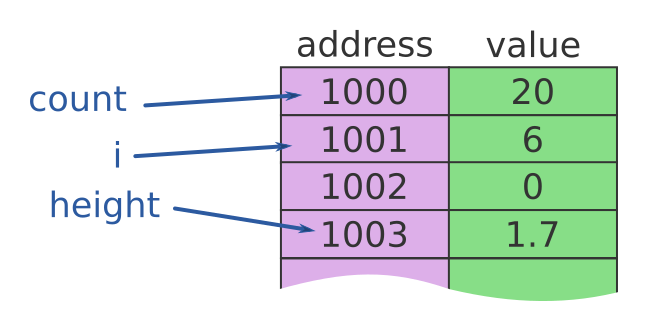# Using variables

Martin McBride, 2017-01-31
Tags none
Categories none

A variable is a place in memory where a value can be stored.

We give each variable a name, so that we can refer to it in our program. Here is an example of some variables stored in memory:Each variable is stored at a particular address in memory, but we don't need to worry about where it is stored, we can access the data using the variable name.

For example, if we wanted to show the value of height we can just use:

PRINT(height)


According to the diagram, the variable is stored at address 1002 in memory, but as programmers we don't need to know or care where it is stored.

## Creating variables

Before we use a variable, we must declare it. Here is how we might declare the 3 variables above, and set their initial values:

INT count = 20
INT i = 6
FLOAT height = 1.7


Declaring the variable count does 3 things:

• It tells the compiler or interpreter that we will be using a variable called count.
• It defines the type of the variable to be an INT (integer), so it can only be used to store integer values. If we later try to store a value 11.9 in count, we will again get an error.
• It sets the value of count to 20.

{{% green-note %}} Declaring the type of a variable when it is created is called static typing. It allows the compiler or interpreter to detect certain errors before the code even runs. {{% /green-note %}}

Not all languages define the type of a variable. In JavaScript, variables are declared with the var keyword, and any variable can hold any type of data. In Python variables don't need to be declared at all - they are automatically created the first time they are used.

{{% yellow-note %}} Python and JavaScript use dynamic typing, which allows any variable to hold any type of data. This can be useful because it allows the same code to work with different types of data. {{% /yellow-note %}}

## Assignment

In maths, when you write

x = y + 3


it is statement of fact, an equality. Whatever the value of x and y, it will always be true that x is 3 bigger than y. If you are told that x is 10, then you can deduce that y must be 7.

In computing, this same statement is called an assignment statement. It represents and instruction - take the current value of variable y, add 3 to it, and place the result in the variable x. For example:

INT x = 2
INT y = 6
x = y + 3


After running this code, the variable x will contain the value 9 (y + 3).Here is another assignment:

x = x + 2


This code simply replaces the current value of x with its value plus 2. So if x had value 8 to start with, it would be 10 after running the code.

Viewed as a mathematical equation, x = x + 2 makes no sense, how can x be equal to x + 2?

{{% blue-note %}} To avoid confusion, some languages use a different symbol for assignment. For example Pascal uses:

x := x + 2


But many languages just use the equal sign, so it is probably best to get used to it! {{% /blue-note %}}

## Constants

The term variable implies that the value of a variable can change as the program runs. And that is usually what happens.

But sometimes you might have a value which shouldn't change while the program is running. For instance, you might want to store the current version of your software, so that you can display it in the splash screen.

We can do this by declaring the version as a constant. A constant is named value which cannot be changed by the code while the program runs. Here is how you declare a constant:

CONST INT VERSION = 4;


Some points to note:

• The CONST keyword prevents the code from changing the value of VERSION after it has been created.
• By giving the constant a name, whenever we use the version value in the code we can see what it means.
• If we need to change the version when we release new software, we only need to change it in one place.
• We normally use UPPER CASE names for constants, so we can tell at a glance that a value is constant. You don't have to, but it is a good idea.

{{% green-note %}} You should try to use constants in your code, it makes it easier to read and understand. Suppose instead of using a constant, we just used the number 4 wherever we needed the version? What happens when you need to change the version to 5? You would have to search through your code for every place that the number 4 appears (probably lots of places) and for each one you would need to read the code to decide is this number 4 the version number, or is it just a 4 which is there for some other reason. {{% /green-note %}}